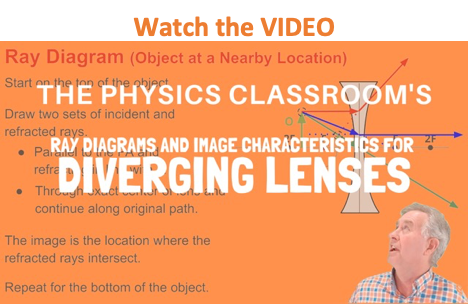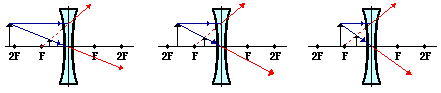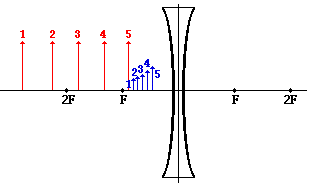Refraction and the Ray Model of Light - Lesson 5 - Image Formation by Lenses

# Diverging Lenses - Object-Image RelationsPreviously in Lesson 5, ray diagrams were constructed in order to determine the location, size, orientation, and type of image formed by double concave lenses (i.e., diverging lenses). The ray diagram constructed earlier for a diverging lens revealed that the image of the object was virtual, upright, reduced in size and located on the same side of the lens as the object. But will these always be the characteristics of an image produced by a double concave lens? Can convex lenses ever produce real images? Inverted images? Magnified Images? To answer these questions, we will look at three different ray diagrams for objects positioned at different locations along the principal axis. The diagrams are shown below. (Note that only two sets of incident and refracted rays were used in the diagram in order to avoid overcrowding the diagram with rays.)The diagrams above show that in each case, the image is

• located on the object' side of the lens
• a virtual image
• an upright image
• reduced in size (i.e., smaller than the object)

Unlike converging lenses, diverging lenses always produce images that share these characteristics. The location of the object does not affect the characteristics of the image. As such, the characteristics of the images formed by diverging lenses are easily predictable.

Another characteristic of the images of objects formed by diverging lenses pertains to how a variation in object distance affects the image distance and size. The diagram below shows five different object locations (drawn and labeled in red) and their corresponding image locations (drawn and labeled in blue).The diagram shows that as the object distance is decreased, the image distance is decreased and the image size is increased. So as an object approaches the lens, its virtual image on the same side of the lens approaches the lens as well; and at the same time, the image becomes larger.

### We Would Like to Suggest ...Why just read about it and when you could be interacting with it? Interact - that's exactly what you do when you use one of The Physics Classroom's Interactives. We would like to suggest that you combine the reading of this page with the use of our Optics Bench Interactive. You can find this in the Physics Interactives section of our website. The Optics Bench Interactive provides the learner an interactive enivronment for exploring the formation of images by lenses and mirrors. Its like having a complete optics toolkit on your screen.

The following questions pertain to the image characteristics of all types of optical devices discussed in the last two units - plane mirrors, concave mirrors, convex mirrors, converging lenses, and diverging lenses. Use your understanding of the object-image relationships for these three types of mirrors and two types of lenses to answer these questions.

1. How can a plane mirror, concave mirror, convex mirror, converging lens and/or diverging lens be used to produce an image that has the same size as the object?

2. How can a plane mirror, concave mirror, convex mirror, converging lens and/or diverging lens be used to produce a magnified image?

3. How can a plane mirror, concave mirror, convex mirror, converging lens and/or diverging lens be used to produce an upright image?

4. How can a plane mirror, concave mirror, convex mirror, converging lens and/or diverging lens be used to produce a real image?

5. The image of an object is found to be upright and reduced in size. What type of mirror and/or lens is used to produce such an image?

Next Section: